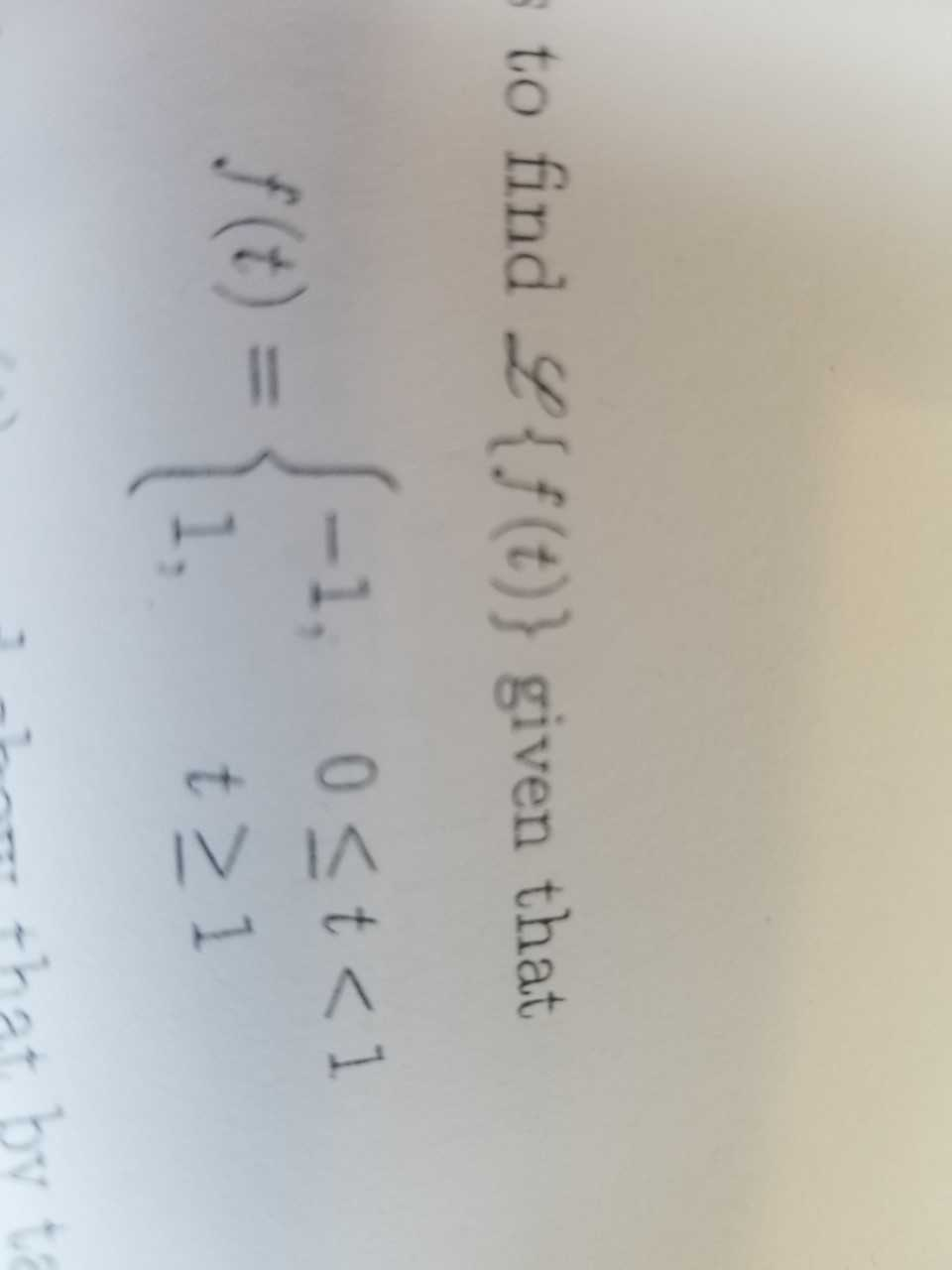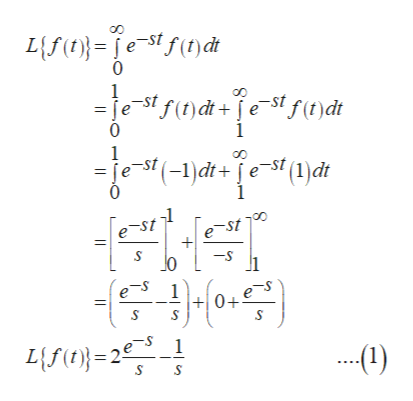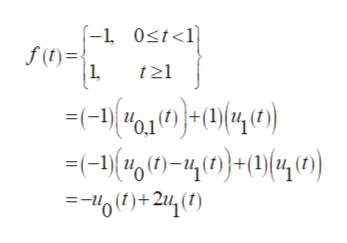s to find {f(t)} given that-1, 0

Question

Use the definition of Laplace Transforms to find l{f(t)}

See image.

Then rewrite f(t) as a sum of step functions, uc(t), and show that by taking Laplace transforms, this yields the same answer as your direct computation.help_outlineImage Transcriptioncloses to find {f(t)} given that -1, 0
Step 1

Consider the given function:

Step 2

Now, by definition of Laplace Transform:help_outlineImage TranscriptioncloseL{f(testf()dt 0 1 =je-st f()dt+est f(t)dt 0 1 1 =je st (-1)dt+e-St (1)dt 0 1 e-st -st0 -S 하045 0+eS 1 Lf(t)=2e fullscreen
Step 3

Now, Rewrite f(t) as the sum of step functions, ...help_outlineImage Transcriptionclose-1 0st<1 f(t) 1 t1 (-1)01(0+1)(4 -(-)(4 )-40)((4(0) =-0()+214() fullscreen

Want to see the full answer?

See Solution

Want to see this answer and more?

Our solutions are written by experts, many with advanced degrees, and available 24/7

See Solution
Tagged in

Math